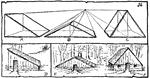### Drawing House Forms

A, B, and C show three types of triangular prisms: right, isosceles, and equilateral. When put together…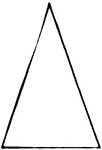### Isosceles

An isosceles triangle is one having two of its sides equal.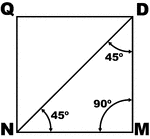### Square

Square with diagonal and angles labeled.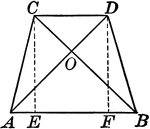### Isosceles Trapezoid With Equal Diagonals

Illustrations of an isosceles trapezoid with equal diagonals drawn.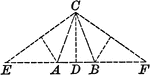### Triangle With Altitude and Isosceles Triangles Within

Illustration showing a triangle with an altitude CD passing through the middle of EF. Triangles AEC…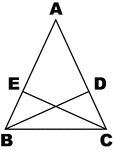### Altitudes Drawn from Base Angles of Isosceles Triangles

Illustration to show altitudes drawn from base angles of an isosceles triangle.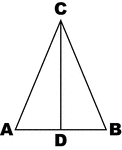### Isosceles Triangle

Sketch of isosceles triangle with labels.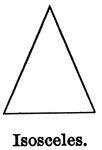### Isosceles Triangle

Illustration showing an isosceles triangle (one that has two sides equal).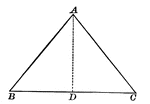### Isosceles Triangle With Interior Segment Drawn

Illustration showing an isosceles triangle with a segment inside. This can be used to show that in an…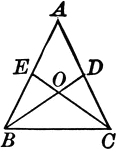### Medians Drawn Drawn to Legs of Isosceles Triangles

Illustration to show the medians drawn to the legs of an isosceles triangle.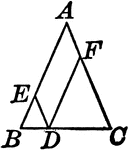### Isosceles Triangle With Parallelogram inside

Illustration to show that if from any point in the base of an isosceles triangle parallels to the legs…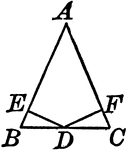### Perpendiculars Dropped From Midpoint of Base to Legs of Isosceles Triangles

Illustration to show the perpendiculars dropped from the midpoint of the base to the legs of an isosceles…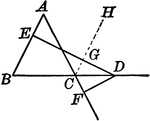### Point in Base of Isosceles Triangle

Illustration to show that the difference of the distances from any point in the base produced of an…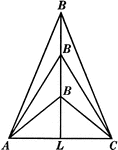### 3 Isosceles Triangles Wit Equal Bases

Illustration of three isosceles triangles with the same base AC but varying heights (as B gets farther…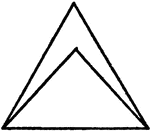### Equilateral And Isosceles Triangles

An illustration showing an isosceles triangle in an equilateral triangle.